# The Development of Mathematical Reasoning

Jun 06, 2020

Check out this exchange I recently had on Twitter. I was discussing frustrations of middle school teachers: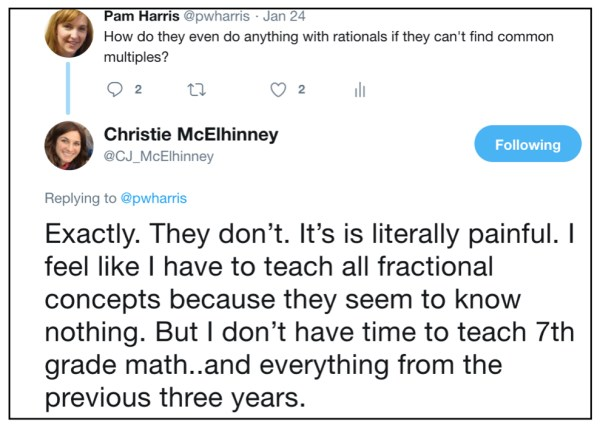Have you ever felt like this Tweet, that you don’t have the time to teach your content and all of the content your students should have learned before you? Why do we have students who seemed to do fine last year, but don’t have what they need to succeed now? How is it that so many students have so many gaps?

• Do you teach middle school and find that your students don’t know their multiplication facts?
• Do you teach algebra and find that your students don’t understand ratio?

I have thought a lot about this. I have thought and researched and read and worked with teachers and students at all grade levels. And I think I have found something that can help.

This post is intended to start that conversation.

I invite you to consider this graphic that represents the development of mathematical reasoning.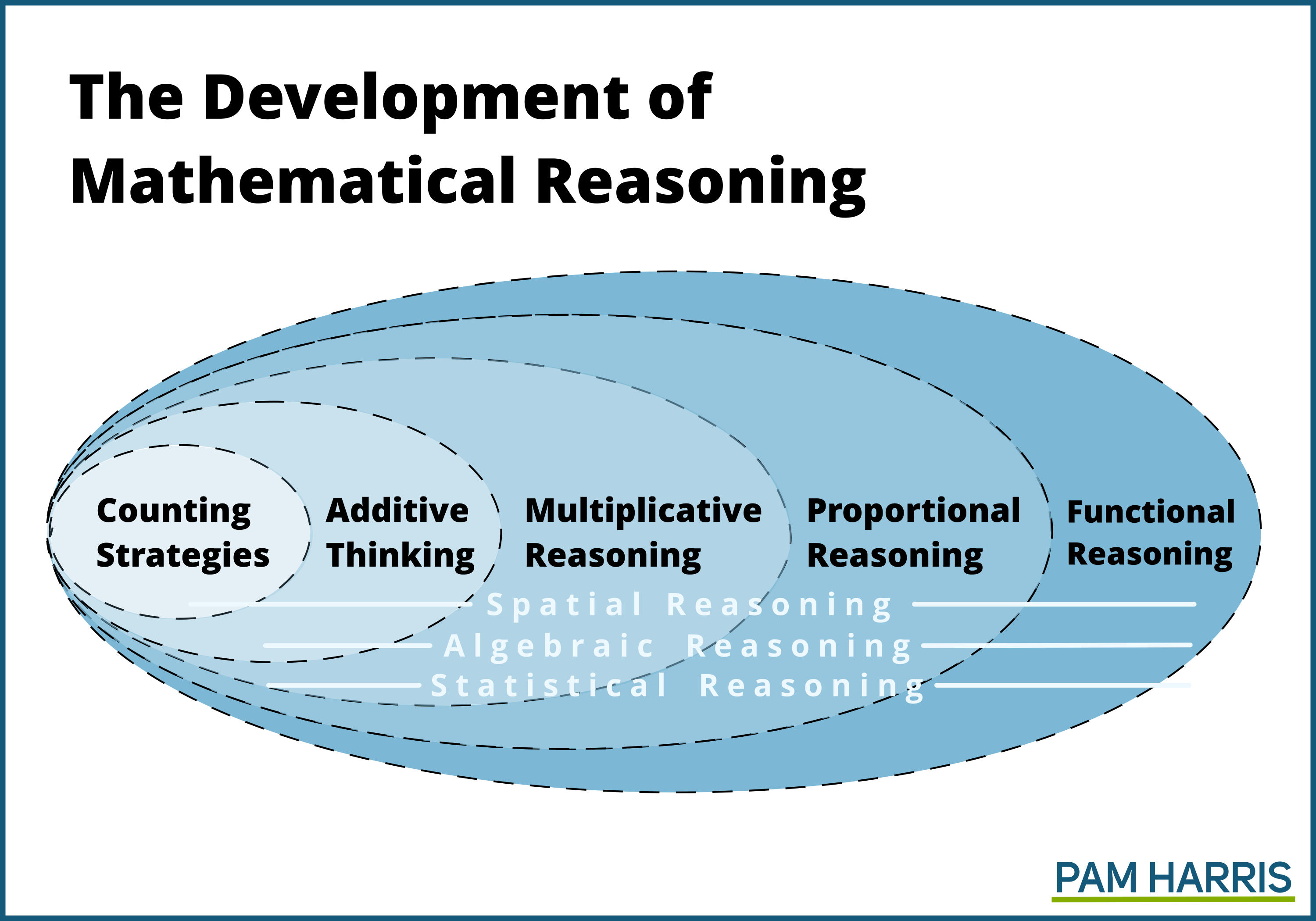Notice how these types of reasoning build on each other.  Each domain of thinking and reasoning is based on the previous and is more complicated, more sophisticated, where more things are happening simultaneously than the previous.

This graphic is meant to suggest that the teacher’s role is to help students change the way they think, in increasingly sophisticated ways. Too often, well-meaning teachers help students get answers to questions, but because it’s easier they often use methods with reasoning in the level(s) before. Students get answers but don’t increase/improve the way they think.  Then students are stymied when they are expected to solve problems in the next level involving more layers of complexity. They haven’t developed the ability to think with sophisticated mathematics, they only know how to get answers.  We, as teachers, need to understand the development of mathematical reasoning so we can help our students think and reason in ways that allow them to continue to grow and learn.

In this post, I’ll give examples of what it means to reason in these increasingly sophisticated ways. For a more in depth study, I also offer a FREE workshop exploring Developing Mathematical Reasoning.

Later on in the post, I’ll give you a helpful one-page questionnaire to help you decide how your students are thinking and reasoning.

#### Counting Strategies

What does it mean to use counting strategies to solve problems?

Counting strategies involve counting by 1s. Here are examples of solving problems involving the four operations counting by 1s.

### Counting Strategies

27 + 8: 27, 28, 29, 30, 31, 32, 33, 34, 35. This could be done with fingers, tally marks, pictures, beads on a rack, on a number line. The part that makes it a counting strategy is the counting by 1s.
27 − 8: Student says 27. Then puts up a finger and says 26, puts up another finger and says 25… puts up an eighth finger and says 19.
7 × 8: Count out 8 (tallies, beans, etc.) into a pile. Then do that 6 more times so you have 7 piles, each with 8 in them. Then start at the beginning and count them all by 1s.
40 ÷ 8: Draw 8 circles. Deal out 40 tallies, one at a time to each circle. Count the number of tallies in each circle: 1, 2, 3, 4, 5.

These are all counting strategies, even though the student is finding answers to addition, subtraction, multiplication, and division problems. The student’s brain is thinking in terms of counting by 1s.

What does it mean to solve problems using additive thinking?

Additive thinking means students solve problems using bigger amounts of numbers than 1 at a time.

27 + 8: 27 + 3 is 30, then add the leftover 5, 35.
27 + 49: 27 and 50 is 77, but that’s a bit too much, so I’ll back up to 76.
27 − 8: 27 subtract 7 is 20, subtract 1 more is 19.
52 − 27: 52 subtract 30 is 22, but I subtracted too much, so adjust back up to 25.
7 × 8: Seven 8s. 8, 16, 24, 32, 40, 48, 56. This is called skip counting but it’s not counting by 1s. It is adding one group of 8 at a time. That’s additive thinking.
48 ÷ 8: 48 − 8 = 40; 40 − 8 = 32; 32 − 8  = 24; 24 − 8 = 16; 16 − 8 = 8. How many 8’s? 6. This is additive thinking, repeatedly subtracting 8s and keeping track of how many 8s.

These are all additive strategies even though in two of these examples, the student is finding answers to multiplication and division problems. If they are doing it one group at a time, that’s additive.

Want an even deeper dive with videos of kids in acton? Check it out here

#### Multiplicative Thinking

What does it mean to solve problems using multiplicative thinking?

Multiplicative thinking means students solve problems using bigger chunks than one group at a time.

### Multiplicative Strategies

7 × 8: I know that seven 7s is 49. But I need eight 7s so I need one more 7. 49 and 7 is 56.
7 × 59: I’m going to think of this as fifty-nine 7s. I know that sixty 7s is 420. But I only need fifty-nine 7s, so 420 − 7 is 413.
48 ÷ 8: I know that 40 ÷ 8 is 5, so 48 ÷ 8 must be 6.
1287 ÷ 13: I know that 1,300 ÷ 13 is 100, but I need 13 less than 1,300, 1287 ÷ 13, so that’s 99.

These are all multiplicative reasoning because the students are working with bigger chunks than one group at a time.

 Solve for x in: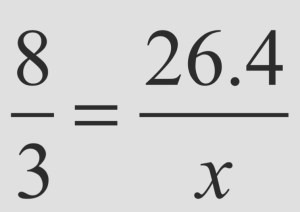Multiply 3 × 26.4 and then divide by 8. This is solving a proportion using multiplicative thinking (although it depends on how you actually think about the multiplication and division–if you just type it in a calculator or perform a rote algorithm, that’s not using multiplicative reasoning.)

Watch students think multiplicatively here

#### Proportional Reasoning

What does it mean to solve problems using proportional reasoning?

Students consider the relationship of two quantities at the same time and the effect of scaling up or down in tandem.

### Strategies using Proportions

Solve for x in:Consider the ratio 8:3 and scaling the 8 to get to 26.4, scaling the 3 along in tandem. 8 × 3 is 24, and 3 × 3 is 9 so now you have equivalent ratios of: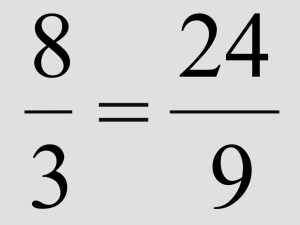Since 8 × 0.3 is 2.4, then you have the equivalent ratios of: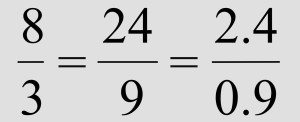and finally: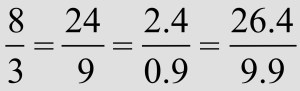This is reasoning in tandem, using equivalent ratios, which is an example of proportional reasoning.

If it takes 3 workers 18 days to do a job, how long will it take 1 worker?  It will take one worker, working alone, three times as long, so 3 × 18 days is 54 days. How long will it take 9 workers? Three times the workers can get the job done in ⅓ the time, so 6 days. This is an example of reasoning inverse proportionally.

These are examples of proportional reasoning because the student recognizes the proportionality and reasons with the ratio in tandem, both parts of the ratio simultaneously.

#### Functional Reasoning

What does it mean to reason using functional reasoning?

It means that students consider the effect of the rate (which is a ratio) on the parent function, which is (often) a set of infinite points that follow a rule. Because they are considering 2 dimensional points, this means that the student is also considering the effect the domain has on the range as it interacts with the rule. Many things are happening simultaneously.

Here are examples of students using functional reasoning.

### Strategies using Functional Reasoning

Find a good viewing window for the function:

=100x2

The student considers a domain of [-10,10] and then uses the function to determine a reasoning range, 100(-10)2 and 100(10)2 for a domain of [10,000, 10,000]. Knowing that the range depends on the chosen domain indicates the simultaneously consideration of the rule, =100x2, the scale factor of 100(rate), and how it impacts an infinite set of points.

Determining the long run behavior of a rational function like: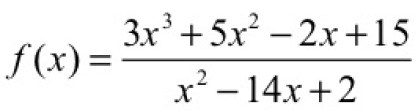The student reasons to find the long run behavior by approximating the quotient of 3x and realizing that the set of points in the extremes will approach the function = 3x. Reasoning that the long run behavior of a rational function will behave the way the ratio of the numerator to the denominator combines proportional reasoning with functional reasoning.

These are examples of functional reasoning because the student simultaneously considers the effect of the ratio (rate) on a relationship of x’s to y’s that create an infinite set of points.

See functional reasoning in action. Check it out here!Close